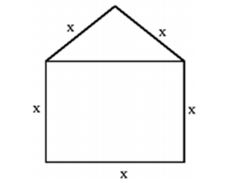Home » Verbal Ability » Numerical Ability » Question

#### Numerical Ability

1. A window is made up of a square portion and an equilateral triangle portion above it. The base of the triangular portion coincides with the upper side of the square. If the perimeter of the window is 6 m, the area of the window in m² is
1. 1.43
2. 2.06
3. 2.68
4. 2.88
##### Correct Option: B

The possible window will be as follows:Perimeter of window = 5x = 6m
x = 1.2 m
So area of window = Area of triangle + Area of square

 = √3 x² + x² 4

 = √3 (1.2)² + (1.2)² 4

= 2.06 m²